# Multivariate Gaussians¶

Modeling Uncertainty in Multiple Dimensions

In :
%matplotlib inline

In :
#format the book
import book_format
book_format.set_style()

Out:

## Introduction¶

The techniques in the last chapter are very powerful, but they only work with one variable or dimension. They provide no way to represent multidimensional data, such as the position and velocity of a dog in a field. Position and velocity are related to each other, and as we learned in the g-h chapter we should never throw away information. In this chapter we learn how to describe this relationship probabilistically. Through this key insight we will achieve markedly better filter performance.

## Multivariate Normal Distributions¶

We've been using Gaussians for a scalar random variable, expressed as $\mathcal{N}(\mu, \sigma^2)$. A more formal term for this is univariate normal, where univariate means 'one variable'. The probability distribution of the Gaussian is known as the univariate normal distribution.

What might a multivariate normal distribution be? Multivariate means multiple variables. Our goal is to be able to represent a normal distribution with multiple dimensions. I don't necessarily mean spatial dimensions - if we track the position, velocity, and acceleration of an aircraft in (x, y, z) that gives us a nine dimensional problem. Consider a two dimensional case. It might be the x and y coordinates of a robot, it might be the position and velocity of a dog on the x-axis, or milk production and feed rate at a dairy. It doesn't really matter. We can see that for $N$ dimensions, we need $N$ means, which we will arrange in a column matrix (vector) like so:

$$\mu = \begin{bmatrix}\mu_1\\\mu_2\\ \vdots \\\mu_n\end{bmatrix}$$

Let's say we believe that $x = 2$ and $y = 17$. We would have

$$\mu = \begin{bmatrix}2\\17\end{bmatrix}$$

The next step is representing our variances. At first blush we might think we would also need N variances for N dimensions. We might want to say the variance for x is 10 and the variance for y is 4, like so.

$$\sigma^2 = \begin{bmatrix}10\\4\end{bmatrix}$$

This is incomplete because it does not consider the more general case. In the Gaussians chapter we computed the variance in the heights of students. That is a measure of how the heights vary relative to each other. If all students are the same height, then the variance is 0, and if their heights are wildly different, then the variance will be large.

There is also a relationship between height and weight. In general, a taller person weighs more than a shorter person. Height and weight are correlated. We want a way to express not only what we think the variance is in the height and the weight, but also the degree to which they are correlated. In other words, we want to know how weight varies compared to the heights. We call that the covariance.

Before we can understand multivariate normal distributions we need to understand the mathematics behind correlations and covariances.

## Correlation and Covariance¶

Covariance describes how much two variables vary together. Covariance is short for correlated variances. In other words, variance is a measure for how a population vary amongst themselves, and covariance is a measure for how much two variables change in relation to each other. For example, as height increases weight also generally increases. These variables are correlated. They are positively correlated because as one variable gets larger so does the other. As the outdoor temperature decreases home heating bills increase. These are inversely correlated or negatively correlated because as one variable gets larger the other variable lowers. The price of tea and the number of tail wags my dog makes have no relation to each other, and we say they are uncorrelated or independent- each can change independent of the other.

Correlation allows prediction. If you are significantly taller than me I can predict that you also weigh more than me. As winter comes I predict that I will be spending more to heat my house. If my dog wags his tail more I don't conclude that tea prices will be changing.

For example, here is a plot of height and weight of students on the school's track team. If a student is 68 inches tall I can predict they weigh roughly 160 pounds. Since the correlation is not perfect neither is my prediction.

In :
from kf_book.gaussian_internal import plot_correlated_data

height = [60, 62, 63, 65, 65.1, 68, 69, 70, 72, 74]
weight = [95, 120, 127, 119, 151, 143, 173, 171, 180, 210]
plot_correlated_data(height, weight, 'Height (in)', 'Weight (lbs)', False)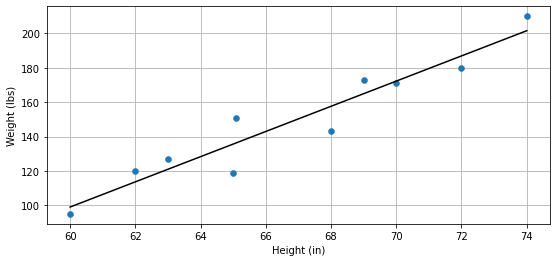In this book we only consider linear correlation. We assume that the relationship between variables is linear. That is, a straight line is a good fit for the data. I've fit a straight line through the data in the above chart. The concept of nonlinear correlation exists, but we will not be using it.

The equation for the covariance between $X$ and $Y$ is

$$COV(X, Y) = \sigma_{xy} = \mathbb E\big[(X-\mu_x)(Y-\mu_y)\big]$$

Where $\mathbb E[X]$ is the expected value of X, defined as

$$\mathbb E[X] = \begin{cases} \sum_{i=1}^n p_ix_i & \mbox{discrete}\\ \int_{-\infty}^\infty f(x)\, x & \mbox{continuous}\end{cases}$$

We assume each data point is equally likely, so the probability of each is $\frac{1}{N}$, giving

$$\mathbb E[X] = \frac{1}{N}\sum_{i=1}^n x_i$$

for the discrete case we will be considering.

Compare the covariance equation to the equation for the variance. As you can see they are very similar:

\begin{aligned}VAR(X) = \sigma_x^2 &= \mathbb E[(X - \mu)^2]\\ COV(X, Y) = \sigma_{xy} &= \mathbb E\big[(X-\mu_x)(Y-\mu_y)\big]\end{aligned}

In particular, if you compute $COV(X, X)$ you get the equation for $VAR(X)$, which supports my statement that the variance computes how a random variable varies amongst itself.

We use a covariance matrix to denote covariances of a multivariate normal distribution, and it looks like this: $$\Sigma = \begin{bmatrix} \sigma_1^2 & \sigma_{12} & \cdots & \sigma_{1n} \\ \sigma_{21} &\sigma_2^2 & \cdots & \sigma_{2n} \\ \vdots & \vdots & \ddots & \vdots \\ \sigma_{n1} & \sigma_{n2} & \cdots & \sigma_n^2 \end{bmatrix}$$

The diagonal contains the variance for each variable, and the off-diagonal elements contain the covariance between the $i^{th}$ and $j^{th}$ variables. So $\sigma_3^2$ is the variance of the third variable, and $\sigma_{13}$ is the covariance between the first and third variables.

A covariance of 0 indicates no correlation. If the variance for $x$ is 10, the variance for $y$ is 4, and there is no linear correlation between $x$ and $y$, then we would write

$$\Sigma = \begin{bmatrix}10&0\\0&4\end{bmatrix}$$

If there was a small amount of positive correlation between $x$ and $y$ we might have

$$\Sigma = \begin{bmatrix}10&1.2\\1.2&4\end{bmatrix}$$

where 1.2 is the covariance between $x$ and $y$. I say the correlation is "small" because the covariance of 1.2 is small relative to the variances of 10.

If there was a large amount of negative correlation between between $x$ and $y$ we might have $$\Sigma = \begin{bmatrix}10&-9.7\\-9.7&4\end{bmatrix}$$

The covariance matrix is symmetric. After all, the covariance between $x$ and $y$ is always equal to the covariance between $y$ and $x$. That is, $\sigma_{xy}=\sigma_{yx}$ for any $x$ and $y$.

I fear I might be losing you, so let's work an example. In the Gaussians chapter we had a class of students with heights H=[1.8, 2.0, 1.7, 1.9, 1.6] meters. We computed:

\begin{aligned} \mathit{VAR}(H) &= E[(H - \mu_H)^2] \\ &= \frac{1}{N}\sum_{i=1}^n (H_i - \mu_H)^2 \\ &= \frac{1}{5}\left[(1.8-1.8)^2 + (2-1.8)^2 + (1.7-1.8)^2 + (1.9-1.8)^2 + (1.6-1.8)^2\right] \\ &= 0.02 \end{aligned}

Easy, right? If we weigh the students we might find their weights to be W = [70.1, 91.2, 59.5, 93.2, 53.5]. Can we use the covariance equation to create the covariance matrix? Sure. It will look like:

$$\Sigma = \begin{bmatrix}\sigma_H^2 & \sigma_{H,W} \\ \sigma_{W,H} & \sigma_{W}^2\end{bmatrix}$$

We just computed the variance of the height, and it will go in the upper left hand corner of the matrix. The lower right corner contains the variance in weights. Using the same equation we get:

\begin{aligned} \mu_W &= \frac{1}{5}(70.1 + 91.2 + 59.5 + 93.2 + 53.5) = 73.5 \\ \sigma_W^2 &= \frac{1}{5}\left[(70.1-73.5)^2 + (91.2-73.5)^2 + (59.5-73.5)^2 + (93.2-73.5)^2 + (53.5-73.5)^2\right] \\ &= 261.8 \end{aligned}

Now the covariances. Using the formula above, we compute:

\begin{aligned} \sigma_{H,W} &= \mathbb E\big[(H-\mu_H)(W-\mu_W)\big] \\ &= \frac{1}{N}\sum_{i=1}^n (H_i-\mu_H)(W_i-\mu_W) \\ &= \frac{1}{5}[(1.8-1.8)(70.1-73.5) + (2-1.8)(91.2-73.5) + (1.7-1.8)(59.5-73.5)\, +\\ &\, \, \, \, \, (1.9-1.8)(93.2-73.5) + (1.6-1.8)(53.5-73.5)] \\ &= 2.18 \end{aligned}

That was tedious, but easy enough. We will never do that again because, of course, NumPy will compute it for you.

In :
import numpy as np

W = [70.1, 91.2, 59.5, 93.2, 53.5]
H = [1.8, 2.0, 1.7, 1.9, 1.6]
np.cov(H, W)

Out:
array([[  0.025,   2.727],
[  2.727, 327.235]])

That doesn't agree with our calculation! What went wrong? Nothing. NumPy applies a correction for small sample sizes; it uses $\frac{1}{N-1}$ as the normalization term instead of $\frac{1}{N}$.

This is a bit beyond the scope of this book. Briefly, suppose the actual class size is 200 students, and we took a sample of 5 students to perform this computation because we couldn't afford to measure and weigh all 200 students. It is nearly certain that there will be some error in our estimator because the sample is unlikely to perfectly represent the class. As our sample size approaches 200 the error will approach 0. We say there is no bias in the latter, and that we have an unbiased estimator. In contrast, when we take a small sample there is bias (error is nonzero), and we have a biased estimator.

If the error is zero it makes sense to divide by $N$. I will not prove why, but for biased estimators we use $\frac{1}{N-1}$ to correct for the small sample size. NumPy does this by default because in practice we are almost always working from data samples from a larger collection. If you want the unbiased estimator, which we computed above, use bias=1 in the call to np.cov'.

In :
np.cov(H, W, bias=1)

Out:
array([[  0.02 ,   2.182],
[  2.182, 261.788]])

This agrees with our computation. We will not use bias=1 again in this book since we are using random variables which are sampling from the infinite set of positions of the objects we are tracking. Here we are computing the variance and covariance for the entire population, so bias=1 is correct.

What does this matrix tell us? It tells us the variance in heights is 0.02 $m^2$ and the variance in weights is 261.788 $kg^2$. Furthermore, it tells us the weights and heights are positively correlated - as heights increase so do the weights.

Let's create perfectly correlated data. By this I mean that the data perfectly fits on a line - there is no variance from the line.

In :
X = np.linspace(1, 10, 100)
Y = np.linspace(1, 10, 100)
np.cov(X, Y)

Out:
array([[6.956, 6.956],
[6.956, 6.956]])

We can see from the covariance matrix that the covariance is equal to the variance in x and in y.

Now let's add some noise to one of the variables so that they are no longer perfectly correlated. I will make $Y$ negative to create a negative correlation.

In :
X = np.linspace(1, 10, 100)
Y = -(np.linspace(1, 5, 100) + np.sin(X)*.2)
plot_correlated_data(X, Y)
print(np.cov(X, Y))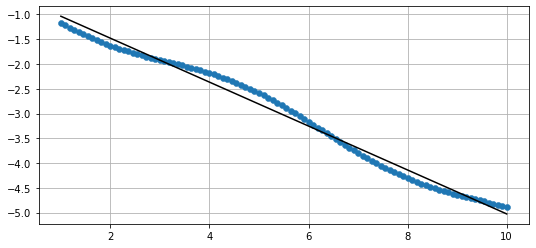[[ 6.956 -3.084]
[-3.084  1.387]]


The data no longer forms a straight line. The covariance is $\sigma_{xy}=-3.08$. It is not close to zero compared to the magnitudes of $\sigma_x^2$ and $\sigma_y^2$, and so we know there is still a high degree of correlation. We can verify this by looking at the chart. The data forms nearly a straight line.

Now I will add random noise to a straight line.

In :
from numpy.random import randn
X = np.linspace(1, 10, 1000) + randn(1000)*2
Y = np.linspace(1, 5, 1000) + randn(1000)
plot_correlated_data(X, Y)
print(np.cov(X, Y))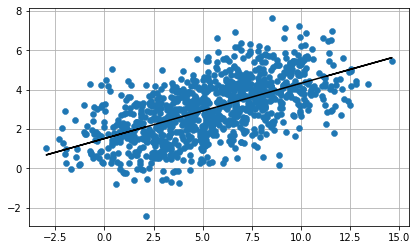[[10.433  2.934]
[ 2.934  2.317]]


We see that the covariance is smaller in relation to the variances, reflecting the lower correlation between $X$ and $Y$. We can still fit a straight line through this data, but there is much greater variation in the data.

Finally, here is the covariance between completely random data.

In :
X = randn(100000)
Y = randn(100000)
plot_correlated_data(X, Y)
print(np.cov(X, Y))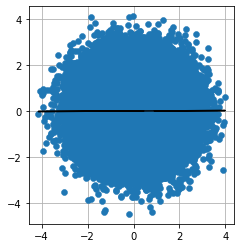[[1.005 0.005]
[0.005 1.005]]


Here the covariances are very near zero. As you can see with the plot, there is no clear way to draw a line to fit the data. A vertical line would be as unconvincing as the horizontal line I've shown.

## Multivariate Normal Distribution Equation¶

Recall the equation for the normal distribution from the Gaussians chapter:

$$f(x, \mu, \sigma) = \frac{1}{\sqrt{2\pi\sigma^2}} \exp \Big [{-\frac{1}{2}}{(x-\mu)^2}/\sigma^2 \Big ]$$

Here is the multivariate normal distribution in $n$ dimensions.

$$f(\mathbf{x},\, \mu,\,\Sigma) = \frac{1}{\sqrt{(2\pi)^n|\Sigma|}}\, \exp \Big [{ -\frac{1}{2}(\mathbf{x}-\mu)^\mathsf{T}\Sigma^{-1}(\mathbf{x}-\mu) \Big ]}$$

The multivariate version merely replaces the scalars of the univariate equations with matrices. If you are reasonably well-versed in linear algebra this equation should look quite manageable. If not, don't worry, both FilterPy and SciPy provide functions to compute it for you. Let's ignore the computation for a moment and plot it to see what it looks like.

In :
import kf_book.mkf_internal as mkf_internal

mean = [2., 17.]
cov = [[10., 0.],
[0., 4.]]

mkf_internal.plot_3d_covariance(mean, cov)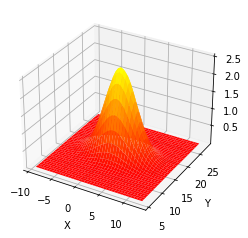This is a plot of multivariate Gaussian with a mean of $\mu=[\begin{smallmatrix}2\\17\end{smallmatrix}]$ and a covariance of $\Sigma=[\begin{smallmatrix}10&0\\0&4\end{smallmatrix}]$. The three dimensional shape shows the probability density for any value of $(X, Y)$ in the z-axis. I have projected the variance for x and y onto the walls of the chart - you can see that they take on the Gaussian bell curve shape. The curve for $X$ is wider than the curve for $Y$, which is explained by $\sigma_x^2=10$ and $\sigma_y^2=4$. The highest point of the 3D surface is at the the means for $X$ and $Y$.

All multivariate Gaussians have this shape. If we think of this as the Gaussian for the position of a dog, the z-value at each point of ($X, Y$) is the probability density of the dog being at that position. Strictly speaking this is the joint probability density function, which I will define soon. So, the dog has the highest probability of being near (2, 17), a modest probability of being near (5, 14), and a very low probability of being near (10, 10). As with the univariate case this is a probability density, not a probability. Continuous distributions have an infinite range, and so the probability of being exactly at (2, 17), or any other point, is 0%. We can compute the probability of being within a given range by computing the volume under the surface with an integral.

FilterPy  implements the equation with the function multivariate_gaussian() in the filterpy.stats. module. SciPy's stats module implements the multivariate normal equation with multivariate_normal(). It implements a 'frozen' form where you set the mean and covariance once, and then calculate the probability density for any number of values for x over any arbitrary number of calls. I named my function multivariate_gaussian() to ensure it is never confused with the SciPy version.

The tutorial for the scipy.stats module explains 'freezing' distributions and other very useful features.

In :
from filterpy.stats import gaussian, multivariate_gaussian


I'll demonstrate using it, and then move on to more interesting things.

First, let's find the probability density for our dog being at (2.5, 7.3) if we believe he is at (2, 7) with a variance of 8 for $x$ and a variance of 3 for $y$.

Start by setting $x$ to (2.5, 7.3). You can use a tuple, list, or NumPy array.

In :
x = [2.5, 7.3]


Next, we set the mean of our belief:

In :
mu = [2.0, 7.0]


Finally, we have to define our covariance matrix. In the problem statement we did not mention any correlation between $x$ and $y$, and we will assume there is none. This makes sense; a dog can choose to independently wander in either the $x$ direction or $y$ direction without affecting the other. I will use the variable name P. Kalman filters use the name $\textbf{P}$ for the covariance matrix, and we need to become familiar with the conventions.

In :
P = [[8., 0.],
[0., 3.]]


Now call the function

In :
%precision 4
multivariate_gaussian(x, mu, P)

Out:
0.0315

We can get the same result from the scipy.stats module.

In :
import scipy
from scipy.stats import multivariate_normal
print(f'{multivariate_normal(mu, P).pdf(x):.4f}')

0.0315


It's time to define some terms. The joint probability, denoted $P(x,y)$, is the probability of both $x$ and $y$ happening. For example, if you roll two die $P(2,5)$ is the probability of the first die rolling a 2 and the second die rolling a 5. Assuming the die are six sided and fair, the probability $P(2,5) = \frac{1}{6}\times \frac{1}{6}=\frac{1}{36}$. The 3D chart above shows the joint probability density function.

The marginal probability is the probability of an event happening without regard of any other event. In the chart above the Gaussian curve drawn to the left is the marginal for $Y$. This is the probability for the dog being at any position in $Y$ disregarding the value for $X$. Earlier I wrote "I have projected the variance for x and y onto the walls of the chart"; these are the marginal probabilities for $x$ and $y$. Another computational benefit of Gaussians is that the marginal of a multivariate Gaussian is another Gaussian!

Let's look at this in a slightly different way. Instead of plotting a surface showing the probability distribution I will generate 1,000 points with the distribution of $[\begin{smallmatrix}8&0\\0&3\end{smallmatrix}]$.

In :
mkf_internal.plot_3d_sampled_covariance(mu, P)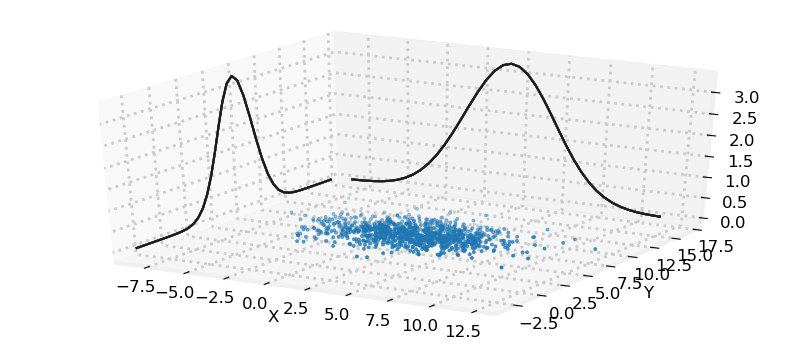We can think of the sampled points as being possible locations for our dog given those particular mean and covariances. The contours on the side show the marginal probability for $X$ and $Y$. We can see that he is far more likely to be at (2, 7) where there are many points, than at (-5, 5) where there are few.

As beautiful as these plots are, it is hard to get useful information from them. For example, it is not easy to tell if $X$ and $Y$ both have the same variance, and how much they are correlated. In most of the book I'll display Gaussians as contour plots.

The contour plots display the range of values that the multivariate Gaussian takes for a specific standard deviation. This is like taking a horizontal slice out of the 3D plot.

These plots show the shape of the slice for 3 standard deviations.

In :
mkf_internal.plot_3_covariances()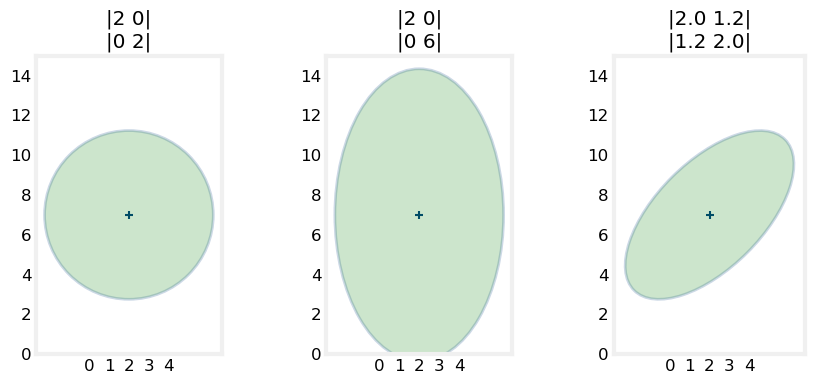For those of you viewing this online or in Juptyer Notebook on your computer, here is an animation of varying the covariance while holding the variance constant.(source: http://git.io/vqxLS)

These plots look like circles and ellipses. Indeed, it turns out that any slice through the multivariate Gaussian is an ellipse. Hence, in statistics we do not call these 'contour plots', but either error ellipses or confidence ellipses; the terms are interchangable.

This code uses the function plot_covariance_ellipse() from filterpy.stats. By default the function displays one standard deviation, but you can use either the variance or std parameter to control what is displayed. For example, variance=3**2 or std=3 would display the 3rd standard deviation, and variance=[1,4,9] or std=[1,2,3] would display the 1st, 2nd, and 3rd standard deviations.

In :
from filterpy.stats import plot_covariance_ellipse
import matplotlib.pyplot as plt

P = [[2, 0], [0, 6]]
plot_covariance_ellipse((2, 7), P, fc='g', alpha=0.2,
std=[1, 2, 3],
title='|2 0|\n|0 6|')
plt.gca().grid(b=False);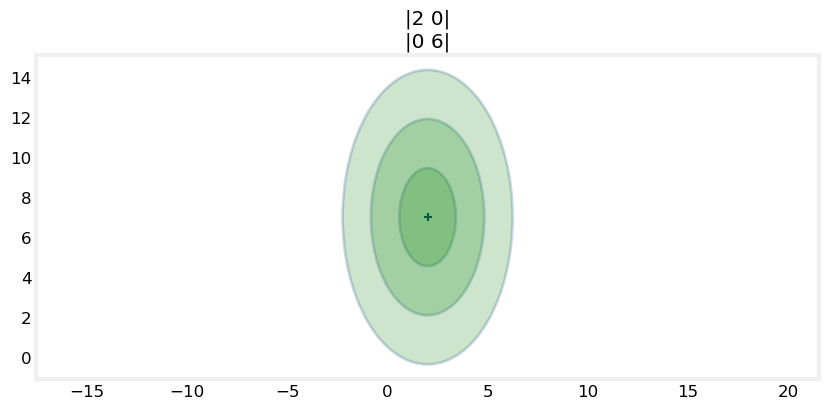The solid colors may suggest to you that the probability distribution is constant between the standard deviations. This is not true, as you can tell from the 3D plot of the Gaussian. Here is a 2D shaded representation of the probability distribution for the covariance ($\begin{smallmatrix}2&1.2\\1.2&1.3\end{smallmatrix})$. Darker gray corresponds to higher probability density.

In :
from kf_book.nonlinear_plots import plot_cov_ellipse_colormap
plot_cov_ellipse_colormap(cov=[[2, 1.2], [1.2, 1.3]]);Thinking about the physical interpretation of these plots clarifies their meaning. The mean and covariance of the first plot is

$$\mathbf{\mu} =\begin{bmatrix}2\\7\end{bmatrix},\, \, \Sigma = \begin{bmatrix}2&0\\0&2 \end{bmatrix}$$

In :
x = [2, 7]
P = [[2, 0], [0, 2]]
plot_covariance_ellipse(x, P, fc='g', alpha=0.2,
title='|2 0|\n|0 2|')
plt.gca().grid(b=False)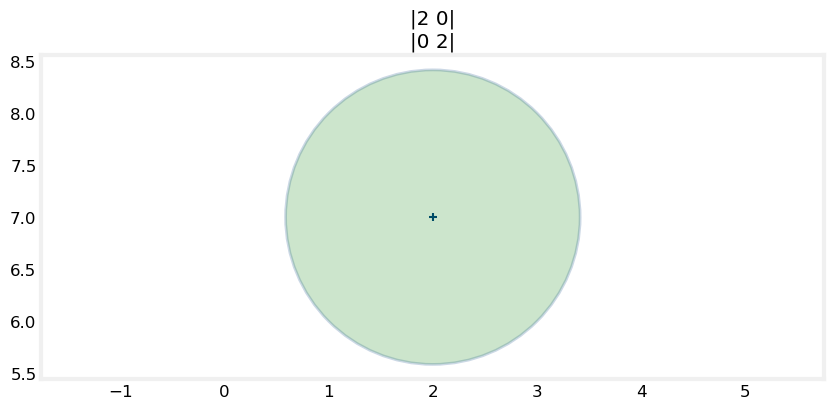A Bayesian way of thinking about this is that the ellipse shows us the amount of error in our belief. A tiny circle would indicate that we have a very small error, and a very large circle indicates a lot of error in our belief. The shape of the ellipse shows us the geometric relationship of the errors in $X$ and $Y$. Here we have a circle so errors in $X$ and $Y$ are equally likely.

The mean and covariance of the second plot are

$$\mu =\begin{bmatrix}2\\7\end{bmatrix}, \, \, \, \Sigma = \begin{bmatrix}2&0\\0&6\end{bmatrix}$$
In :
x = [2, 7]
P = [[2, 0], [0, 6]]
plot_covariance_ellipse(x, P, fc='g', alpha=0.2,
title='|2 0|\n|0 6|')
plt.gca().grid(b=False)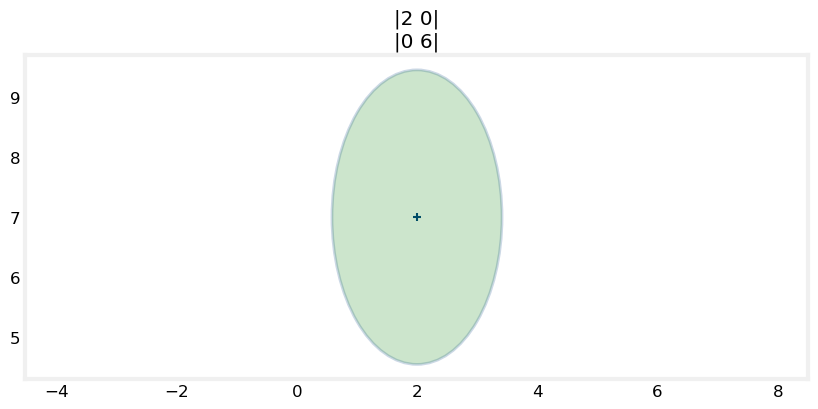This time we use a different variance for $X$ ($\sigma_x^2=2$) vs $Y$ ($\sigma^2_y=6$). The result is a tall and narrow ellipse. We can see that a lot more uncertainty in $Y$ vs $X$. In both cases we believe the dog is at (2, 7), but the uncertainties are different.

The third plot shows the mean and covariance

$$\mu =\begin{bmatrix}2\\7\end{bmatrix}, \, \, \, \Sigma = \begin{bmatrix}2&1.2\\1.2&2\end{bmatrix}$$
In :
x = [2, 7]
P = [[2, 1.2], [1.2, 2]]
plot_covariance_ellipse(x, P, fc='g', alpha=0.2,
title='|2 1.2|\n|1.2 2|')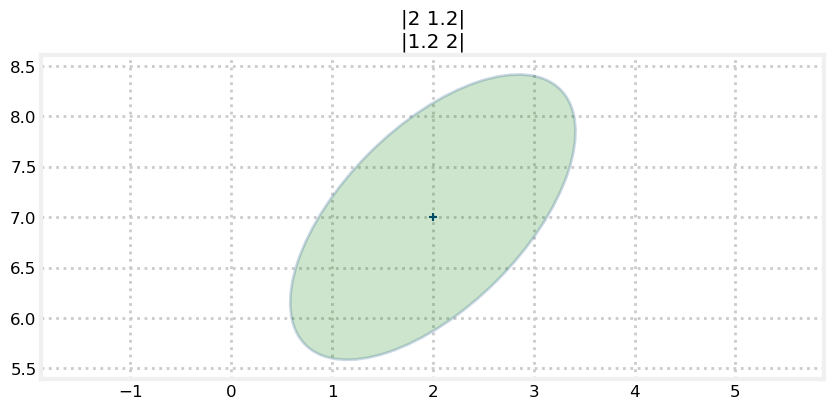This is the first contour that has values in the off-diagonal elements of the covariance, and this is the first contour plot with a slanted ellipse. This is not a coincidence. The two facts are telling us the same thing. A slanted ellipse tells us that the $x$ and $y$ values are somehow correlated. The off-diagonal elements in the covariance matrix are non-zero, indicating that a correlation exists.

Recall the plot for height versus weight. It formed a slanted grouping of points. We can use NumPy's cov() function to compute the covariance of two or more variables by placing them into a 2D array. Let's do that, then plot the $2\sigma$ covariance ellipse on top of the data. We will need to use bias=1 because the data represents the entire population; it is not a sample.

In :
cov_hw = np.cov(np.vstack((height, weight)), bias=1)
cov_hw

Out:
array([[  18.5249,  135.701 ],
[ 135.701 , 1092.29  ]])
In :
plt.scatter(height, weight, s=120, marker='s')
plt.title('Track Team Height vs. Weight')
plt.xlabel('Height (in)'); plt.ylabel('Weight (lbs)')
plot_covariance_ellipse((np.mean(height), np.mean(weight)), cov_hw, fc='g',
alpha=0.2, axis_equal=False, std=2)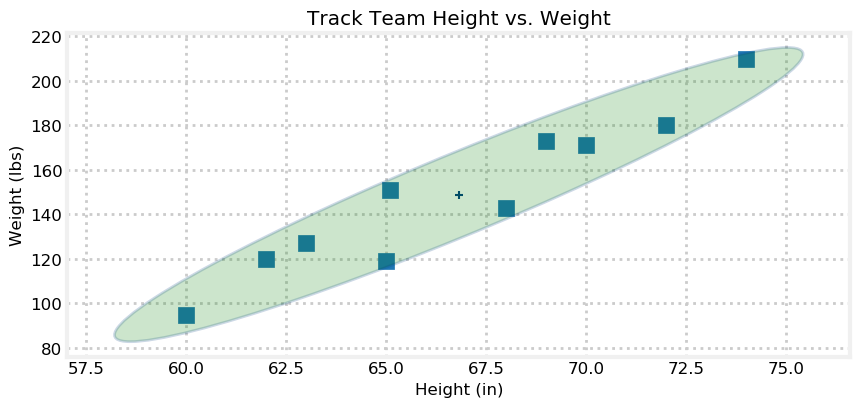This should help you form a strong intuition on the meaning and use of covariances. The covariance ellipse shows you how the data is 'scattered' in relation to each other. A narrow ellipse like this tells you that the data is very correlated. There is only a narrow range of weights for any given height. The ellipse leans towards the right, telling us there is a positive correlation - as x increases y also increases. If the ellipse leaned towards the left then the correlation would be negative - as x increases y decreases. We can see this in the following plot:

In :
max_temp = [200, 250, 300, 400, 450, 500]
lifespan = [10, 9.7, 5, 5.4, 4.3, 0.3]

plt.scatter(max_temp, lifespan, s=80)
cov = np.cov(np.vstack((max_temp, lifespan)))
plot_covariance_ellipse((np.mean(max_temp), np.mean(lifespan)), cov, fc='g',
alpha=0.2, axis_equal=False, std=2)
plt.title('Engine Temperature vs Lifespan')
plt.xlabel('Temperature (C)'); plt.ylabel('Years');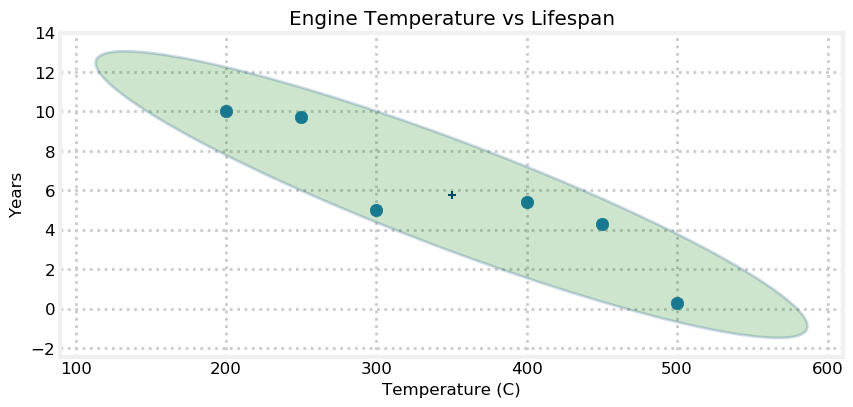The relationships between variances and covariances can be hard to puzzle out by inspection, so here is an interactive plot. (If you are reading this in a static form instructions to run this online are here: https://git.io/vza7b)

In :
from ipywidgets import interact
from kf_book.book_plots import figsize, FloatSlider

fig = None
def plot_covariance(var_x, var_y, cov_xy):
global fig
if fig: plt.close(fig)
fig = plt.figure(figsize=(4,4))
P1 = [[var_x, cov_xy], [cov_xy, var_y]]

plot_covariance_ellipse((10, 10), P1, axis_equal=False,
show_semiaxis=True)

plt.xlim(4, 16)
plt.gca().set_aspect('equal')
plt.ylim(4, 16)

with figsize(y=6):
interact (plot_covariance,
var_x=FloatSlider(5, min=0, max=20),
var_y=FloatSlider(5, min=0, max=20),
cov_xy=FloatSlider(1.5, min=0, max=50, step=.2));


### Pearson's Correlation Coefficient¶

We will not be using this coefficient in this book, but you may see it elsewhere. You can safely skip this section if uninterested.

The correlation between two variables can be given a numerical value with Pearson's Correlation Coefficient. It is defined as

$$\rho_{xy} = \frac{COV(X, Y)}{\sigma_x \sigma_y}$$

This value can range in value from -1 to 1. If the covariance is 0 than $\rho=0$. A value greater than 0 indicates that the relationship is a positive correlation, and a negative value indicates that there is a negative correlation. Values near -1 or 1 indicate a very strong correlation, and values near 0 indicate a very weak correlation.

Correlation and covariance are very closely related. Covariance has units associated with it, and correlation is a unitless ratio. For example, for our dog $\sigma_{xy}$ has units of meters squared.

We can use scipy.stats.pearsonr function to compute the Pearson coefficient. It returns a tuple of the Pearson coefficient and of the 2 tailed p-value. The latter is not used in this book. Here we compute $\rho$ for height vs weight of student athletes:

In :
from scipy.stats import pearsonr
pearsonr(height, weight)

Out:
0.9539731096080194

Here we compute the correlation between engine temperature and lifespan.

In :
pearsonr(max_temp, lifespan)

Out:
-0.9178223453527254

## Using Correlations to Improve Estimates¶

Suppose we believe our dog is at position (5, 10) with some given covariance. If the standard deviation in x and y is each 2 meters, but they are strongly correlated, the covariance contour would look something like this.

In :
P = [[4, 3.9], [3.9, 4]]

plot_covariance_ellipse((5, 10), P, ec='k', std=[1, 2, 3])
plt.xlabel('X')
plt.ylabel('Y');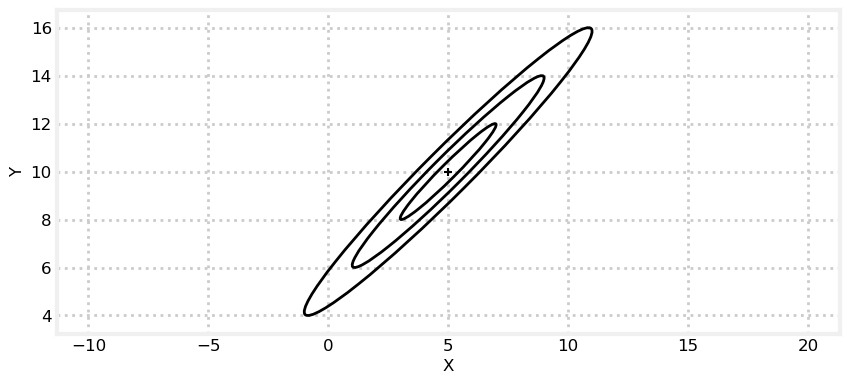Now suppose I were to tell you that we know that $x=7.5$. What can we infer about the value for $y$? The position is extremely likely to lie within the 3$\sigma$ covariance ellipse. We can infer the position in y based on the covariance matrix because there is a correlation between x and y. I've illustrated the likely range of values for y as a blue filled circle.

In :
mkf_internal.plot_correlation_covariance()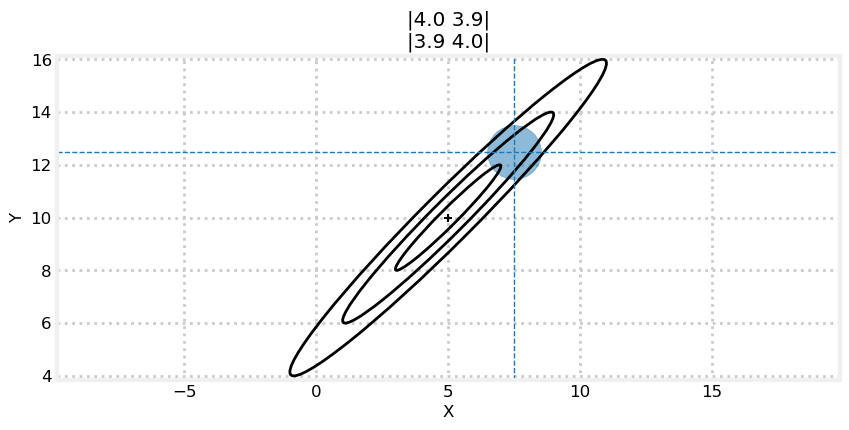The circle not mathematically correct, but it gets the idea across. We will tackle the mathematics in the next section. For now recognize that we can predict that $y$ is likely near 12. A value of $y=-10$ is extremely improbable.

A word about correlation and independence. If variables are independent they can vary separately. If you walk in an open field, you can move in the $x$ direction (east-west), the $y$ direction(north-south), or any combination thereof. Independent variables are always also uncorrelated. Except in special cases, the reverse does not hold true. Variables can be uncorrelated, but dependent. For example, consider $y=x^2$. Correlation is a linear measurement, so $x$ and $y$ are uncorrelated. However, $y$ is dependent on $x$.

## Multiplying Multidimensional Gaussians¶

In the previous chapter we incorporated an uncertain measurement with an uncertain estimate by multiplying their Gaussians together. The result was another Gaussian with a smaller variance. If two pieces of uncertain information corroborate each other we should be more certain in our conclusion. The graphs look like this:

In :
mkf_internal.plot_gaussian_multiply()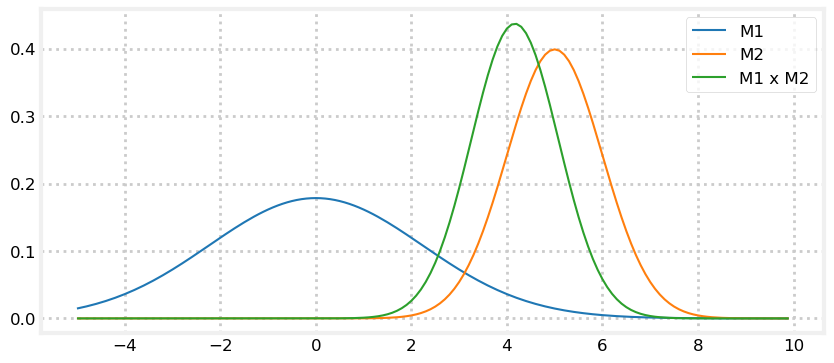The combination of measurements 1 and 2 yields more certainty, so the new Gaussian is taller and narrower - the variance became smaller. The same happens in multiple dimensions with multivariate Gaussians.

Here are the equations for multiplying multivariate Gaussians. The capital sigma ($\Sigma$) indicates that these are matrices, not scalars. Specifically, they are covariance matrices:

\begin{aligned} \mu &= \Sigma_2(\Sigma_1 + \Sigma_2)^{-1}\mu_1 + \Sigma_1(\Sigma_1 + \Sigma_2)^{-1}\mu_2 \\ \Sigma &= \Sigma_1(\Sigma_1+\Sigma_2)^{-1}\Sigma_2 \end{aligned}

They are generated by plugging the multivariate Gaussians for the prior and the estimate into Bayes Theorem. I gave you the algebra for the univariate case in the Gaussians chapter.

You will not need to remember these equations as they are computed by Kalman filter equations that will be presented shortly. This computation is also available in FilterPy using the multivariate_multiply() method, which you can import from filterpy.stats.

\begin{aligned} \mu &=\frac{\sigma_1^2 \mu_2 + \sigma_2^2 \mu_1} {\sigma_1^2 + \sigma_2^2}, \\ \sigma^2 &= \frac{\sigma_1^2\sigma_2^2}{\sigma_1^2+\sigma_2^2} \end{aligned}

This looks similar to the equations for the multivariate equations. This will be more obvious if you recognize that matrix inversion, denoted by the -1 power, is like a reciprocal since $AA^{-1} =I$. I will rewrite the inversions as divisions - this is not a mathematically correct thing to do as division for matrices is not defined, but it does help us compare the equations.

\begin{aligned} \mu &\approx \frac{\Sigma_2\mu_1 + \Sigma_1\mu_2}{\Sigma_1 + \Sigma_2} \\ \\ \Sigma &\approx \frac{\Sigma_1\Sigma_2}{(\Sigma_1+\Sigma_2)} \end{aligned}

In this form the relationship between the univariate and multivariate equations is clear.

Now let's explore multivariate Gaussians in terms of a concrete example. Suppose that we are tracking an aircraft with two radar systems. I will ignore altitude so I can use two dimensional plots. Radar provides the range and bearing to a target. We start out being uncertain about the position of the aircraft, so the covariance, which is our uncertainty about the position, might look like this. In the language of Bayesian statistics this is our prior.

In :
P0 = [[6, 0], [0, 6]]
plot_covariance_ellipse((10, 10), P0, fc='y', alpha=0.6)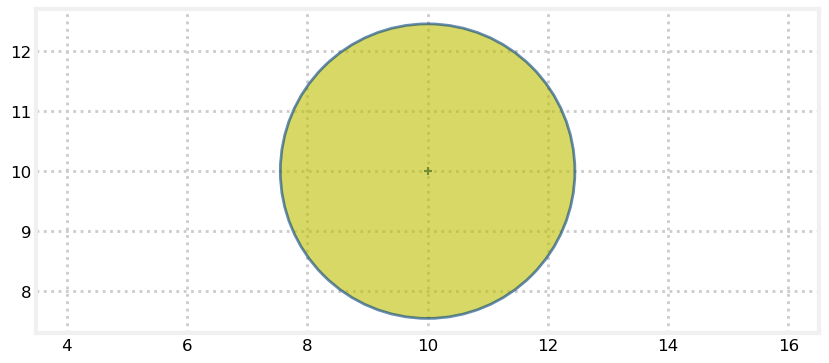Now suppose that there is a radar to the lower left of the aircraft. Further suppose that the radar's bearing measurement is accurate, but the range measurement is inaccurate. The covariance for the error in the measurement might look like this (plotted in green on top of the yellow prior):

In :
P1 = [[2, 1.9], [1.9, 2]]
plot_covariance_ellipse((10, 10), P0, fc='y', alpha=0.6)
plot_covariance_ellipse((10, 10), P1, fc='g', alpha=0.9)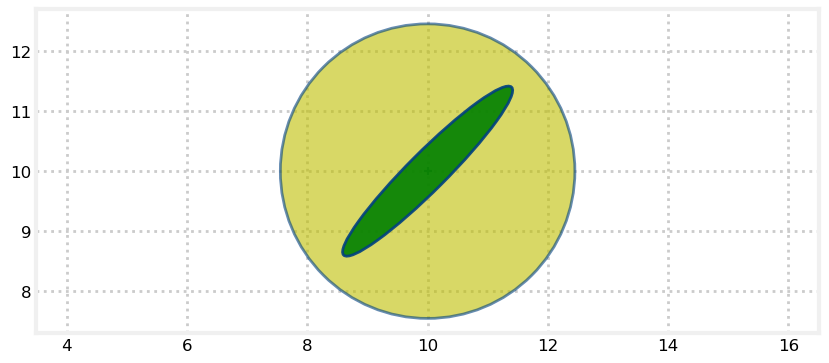Recall that Bayesian statistics calls this the evidence. The ellipse points towards the radar. It is very long because the range measurement is inaccurate, and the aircraft could be within a considerable distance of the measured range. It is very narrow because the bearing estimate is very accurate and thus the aircraft must be very close to the bearing estimate.

We want to find the posterior - the mean and covariance that results from incorporating the evidence into the prior. As in every other chapter we combine evidence by multiplying them together.

In :
from filterpy.stats import multivariate_multiply

P2 = multivariate_multiply((10, 10), P0, (10, 10), P1)
plot_covariance_ellipse((10, 10), P0, ec='k', fc='y', alpha=0.2)
plot_covariance_ellipse((10, 10), P1, ec='k', fc='g', alpha=0.9)
plot_covariance_ellipse((10, 10), P2, ec='k', fc='b')
`